# 6.1   Representing Information

Everything suited for processing with digital computers is represented as a sequence of 0s and 1s, whether it be numeric data, text, executable files, images, audio, or video. The meaning of a given sequence of bits within a computer depends on the context. In this section we describe how to represent integers in binary, decimal, and hexadecimal and how to convert between different representations. We also describe how to represent negative integers and floating-point numbers.

Since Babylonian times, people have represented integers using positional notation with a fixed base. The most familiar of these systems is decimal, where the base is 10 and each positive integer is represented as a string of digits between 0 and 9. Specifically, Specifically, $$d_n d_{n-1} \ldots d_2 d_1 d_0$$ represents the integer
$$\quad\quad\quad\quad\quad\;\; d_n10^n + d_{n-1}10^{n-1} + \ldots + d_210^2 + d_110^1 + d_010^0$$
For example, 10345 represents the integer 10,345 = 1·104 + 0·103 + 3·102 + 4·101 + 5·100.
• Binary. When the base is 2, we represent an integer as a sequence of 0s and 1s. In this case, we refer to each binary (base 2) digit—either 0 or 1—as a bit. Specifically, $$b_n b_{n-1} \ldots b_2 b_1 b_0$$ represents the integer
$$\quad\quad\quad\quad b_n 2^n + b_{n-1} 2^{n-1} + \ldots + b_2 2^2 + b_1 2^1 + b_0 2^0$$
For example, 1100011 represents the integer 99 = 1·26 + 1·25 + 0·24 + 0·23 + 0·22 + 1·21 + 1·20.
• Hexadecimal.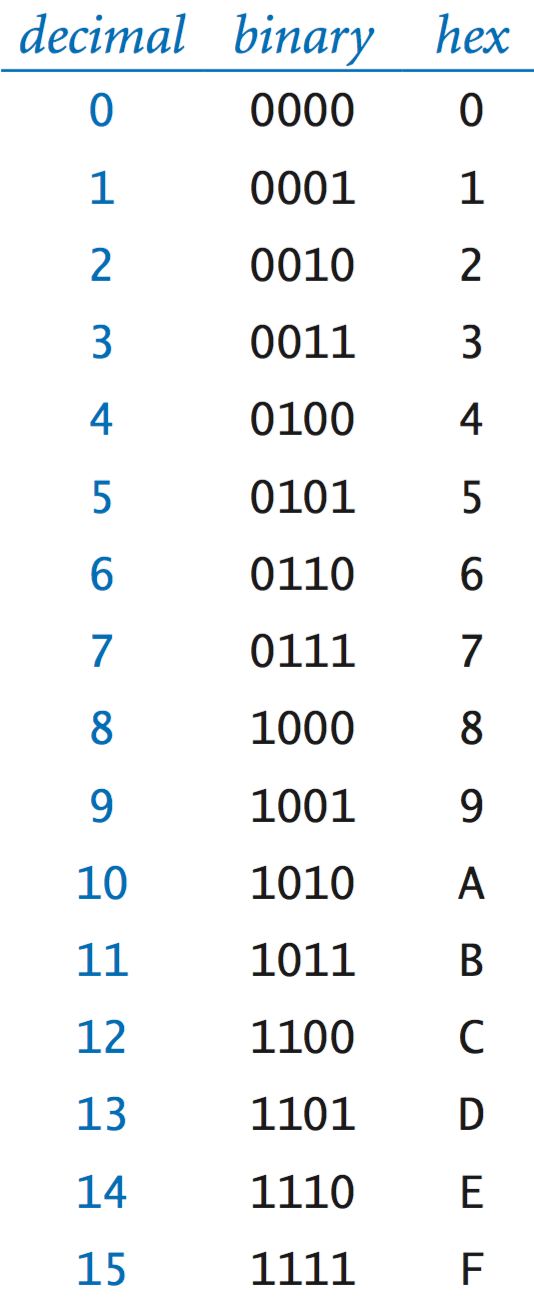In hexadecimal (or hex), the sequence of hex digits Specifically, $$h_n h_{n-1} \ldots h_2 h_1 h_0$$ represents the integer
$$\quad\quad\quad\quad\;\; h_n 16^n + h_{n-1} 16^{n-1} + \ldots + h_2 16^2 + h_1 16^1 + h_0 16^0$$
We need to have one character to represent each digit, so we use A for 10, B for 11, C for 12, and so forth. For example, FACE represents the integer 64,206 = 15·163 + 10·162 + 12·161 + 14·160.

## Number conversion.

You need to know how to convert from a number represented in one system to another.
• Converting between hex and binary. Given the hex representation of a number, finding the binary representation is easy, and vice versa, because 16 is a power of 2. To convert from hex to binary, replace each hex digit by the four binary bits corresponding to its value. Conversely, to convert from binary to hex, prepend leading 0s to make the number of bits a multiple of 4, then group the bits 4 at a time and convert each group to a single hex digit.• Converting from decimal to base b. It is slightly more difficult to convert an integer represented in decimal to one in base b because we are accustomed to performing arithmetic in base 10. The easiest way to convert from decimal to base b by hand is to repeatedly divide by the base b, and read the remainder upwards. For example, the calculations below convert from the decimal integer 366 to binary (101101110) and to hexadecimal (16E).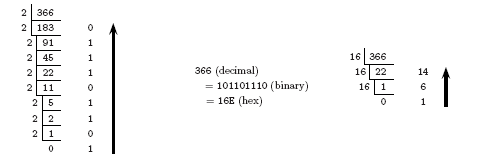• Parsing and string representation. Converting a string of characters to an internal representation is called parsing. First, we consider a method parseInt() to parse integers written in any base. Next, we consider a toString() method to compute a string representation of an integer in any given base. BinaryConverter.java provides methods for converting from a string of bits to a Java int and vice versa. Converter.java is a more general version that handles strings of digits in any base between 2 and 36. These are simplified versions of Java's two-argument Integer.toString() and Integer.parseInt() methods.
The following table contains the decimal, 8-bit binary, and 2-digit hex representations of the integers from 0 to 255.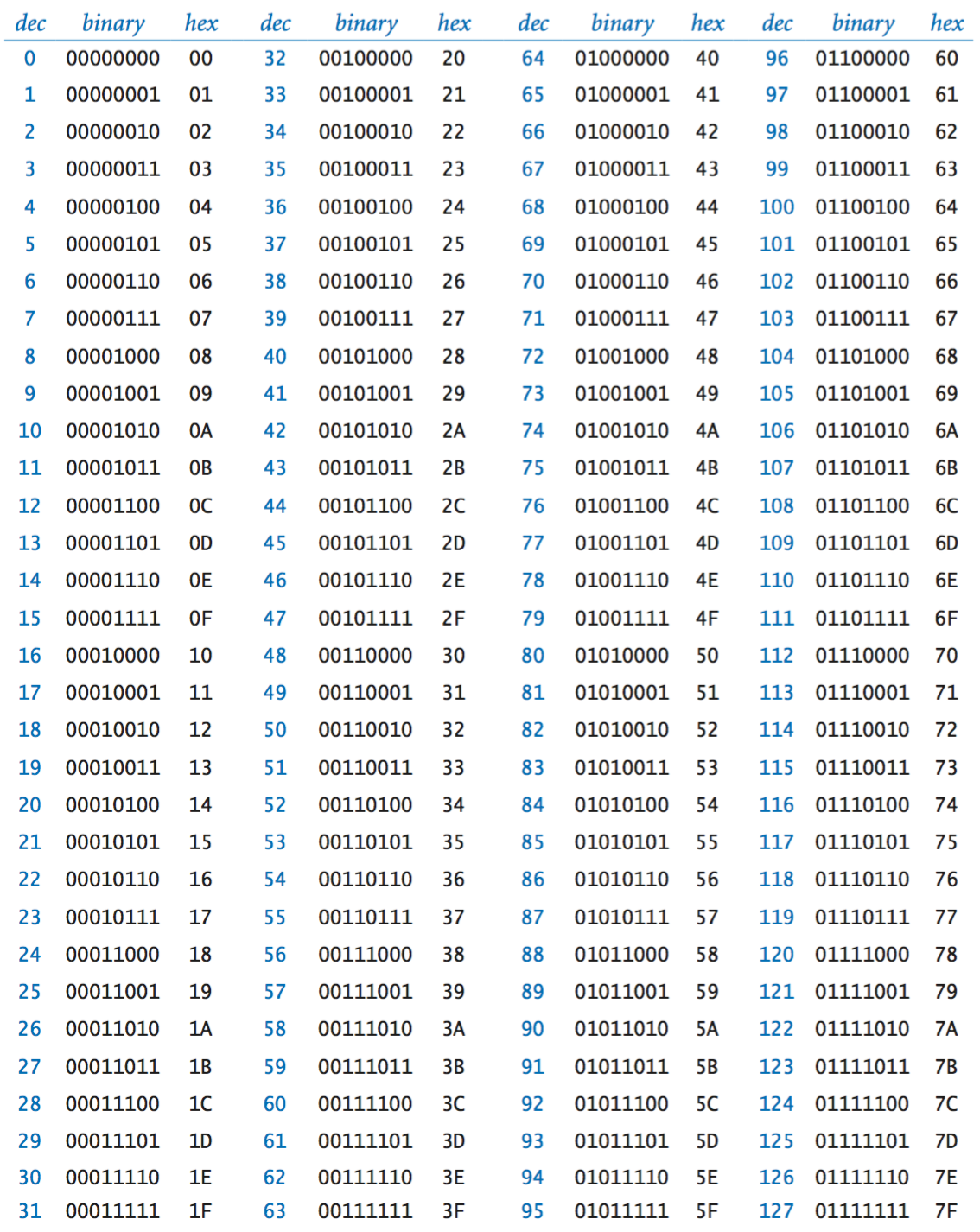## Integer arithmetic.

The first operations that we consider on integers are basic arithmetic operations like addition and multiplication.
• Addition. In grade school you learned how to add two decimal integers: add the two least significant digits (rightmost digits); if the sum is more than 10, then carry a 1 and write down the sum modulo 10. Repeat with the next digit, but this time include the carry bit in the addition. The same procedure generalizes to any base by replacing 10 with the desired base.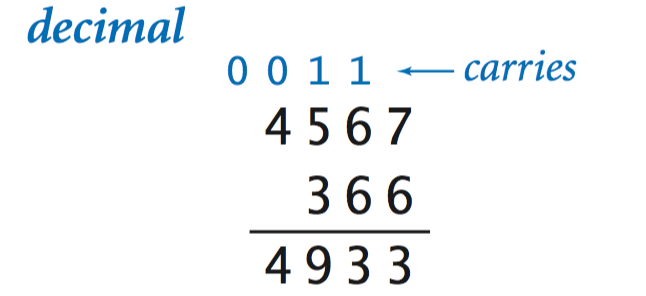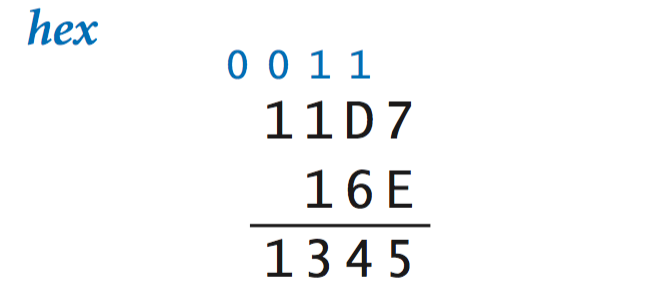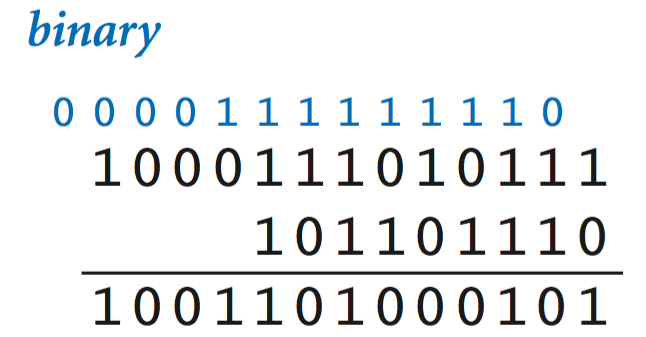• Unsigned integers. We can represent only 2n integers in an n-bit word. If we want just non-negative (or unsigned) integers, the natural choice is to use binary for the integers 0 through 2n − 1, with leading 0s. For example, with 16-bit words, we can represent the integers from 0 to 65,535.
• Overflow. Wee need to pay attention to ensure that the value of the result of an arithmetic operation does not exceed the maximum possible value. This condition is called overflow. For addition of unsigned integers, overflow is easy to detect: if the last (leftmost) addition causes a carry, then the result is too large to represent.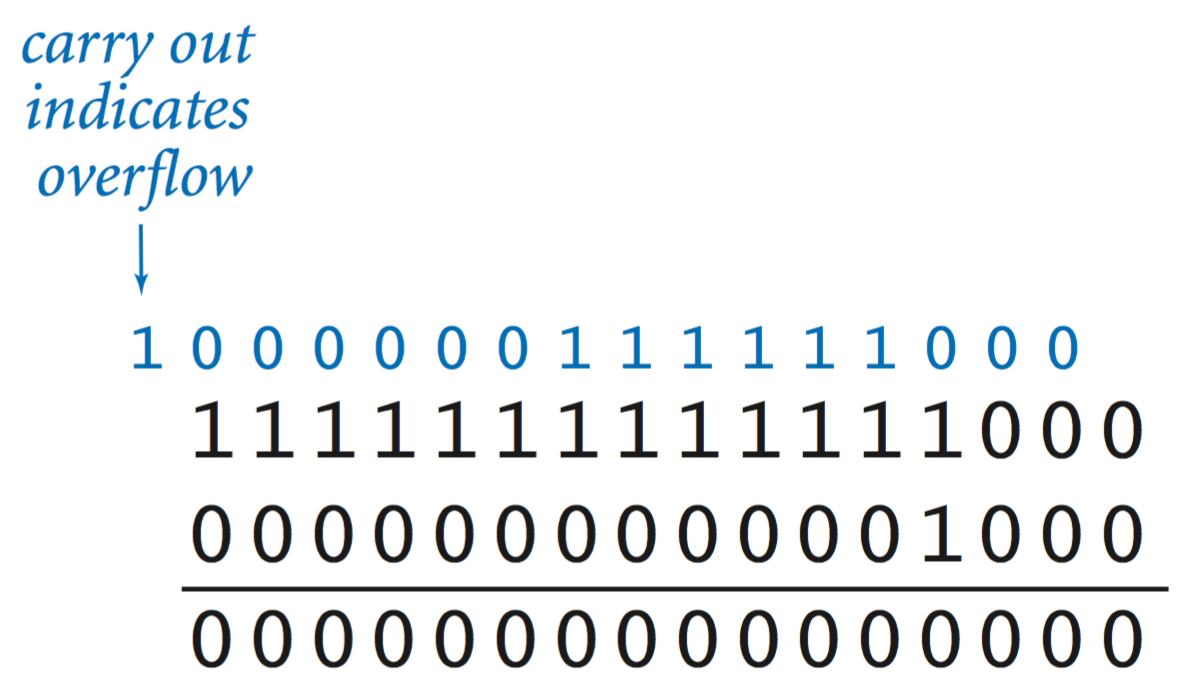• Multiplication. The grade-school algorithm for multiplication works perfectly well with any base.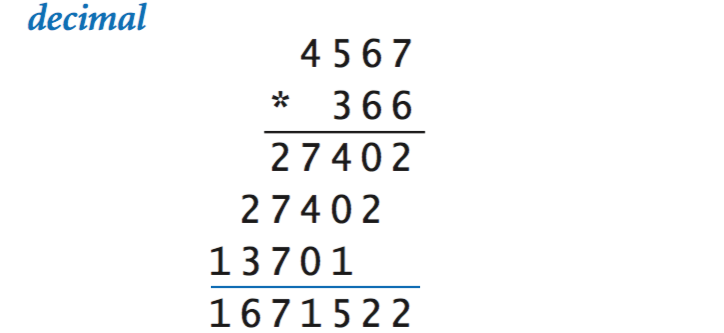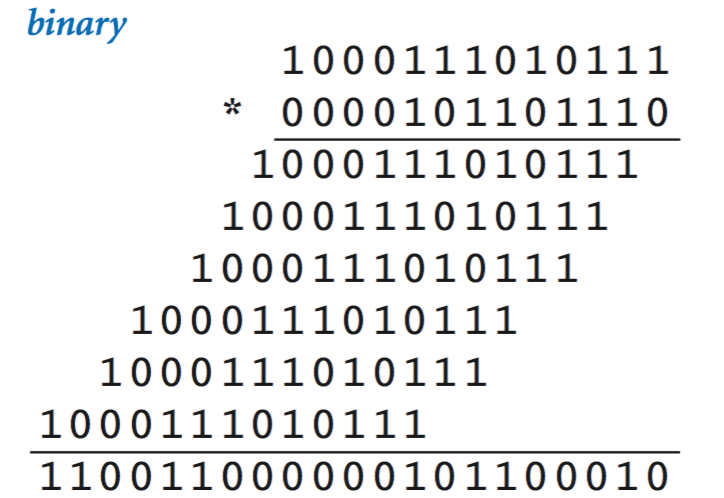## Negative integers.

It is not difficult to modify the integer data type to include negative numbers, using a representation known as two’s complement. In n-bit two’s complement, we represent positive numbers as before, but we represent each negative number –x with the (positive, unsigned) binary number 2nx. For example, the 4-bit two's complement integer 0101 still represents +5 but 1011 represents –5, because 24 – 5 = 1110 = 10112.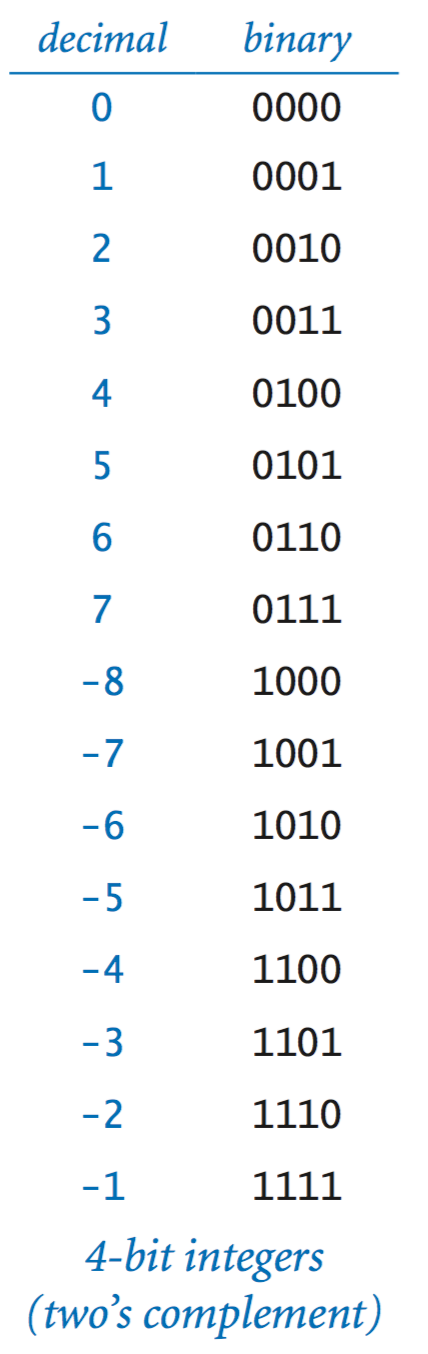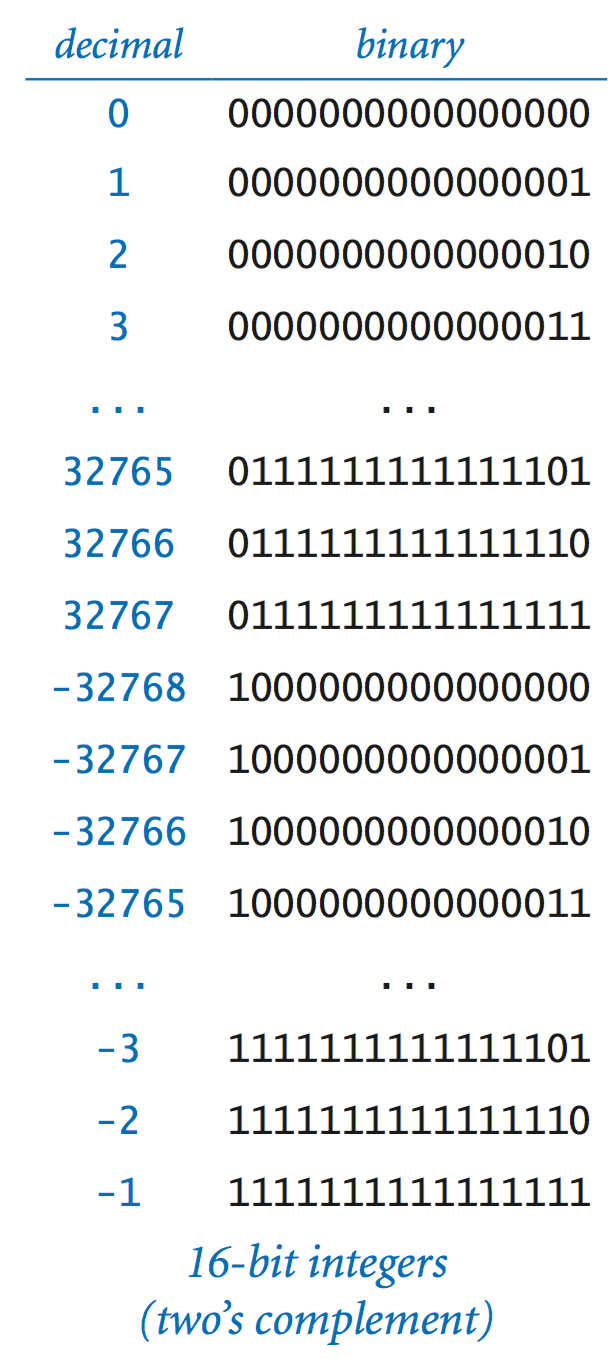• Addition. Adding two n-bit two’s complement integers is easy: add them as if they were unsigned integers. Detecting overflow is a bit more complicated than for unsigned integers.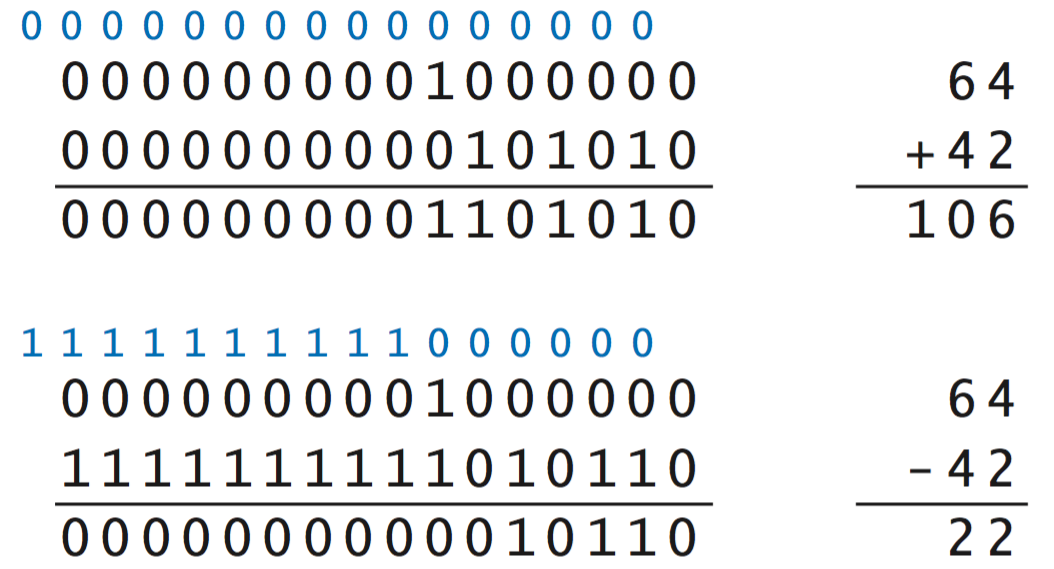• Subtraction. To compute x – y, we compute x + (– y). That is, we can still use standard binary addition, if we know how to compute –y. To negate a two's complement integer, flip the bits and then add 1.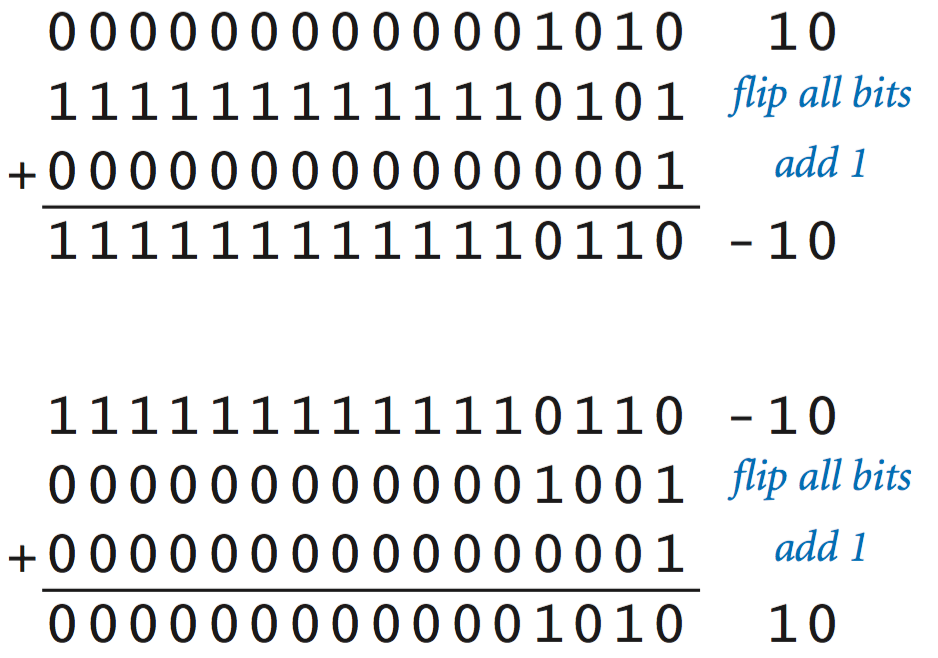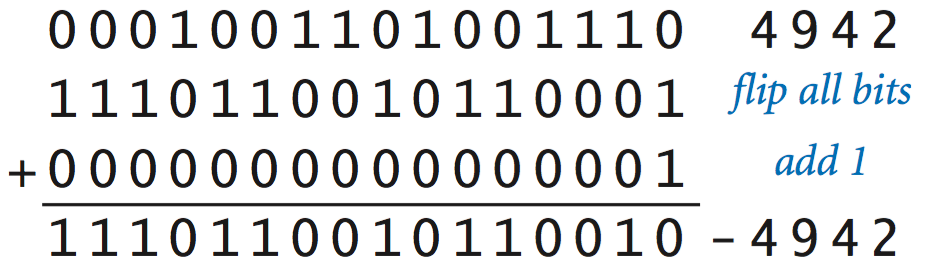• Java. Java's short, int, and long data types are 16-, 32-, and 64-bit two’s complement integers, respectively. This explains the bounds on values of these types and explains the behavior on overflow in Java that we first observed in Section 1.2.

## Real numbers.

The IEEE 754 standard defines the behavior of floating-point number of most computer systems. For simplicity, we illustrate with a 16-bit version known as half-precision binary floating point or binary16 for short. The same essential ideas apply to the 32-bit and 64-bit versions used in Java, which we refer to as binary32 and binary64.
• Floating-point. The real-number representation that is commonly used in computer systems is known as floating point. It is just like scientific notation, except that everything is represented in binary. Just as in scientific notation, a floating-point number consists of a sign, a coefficient, and an exponent.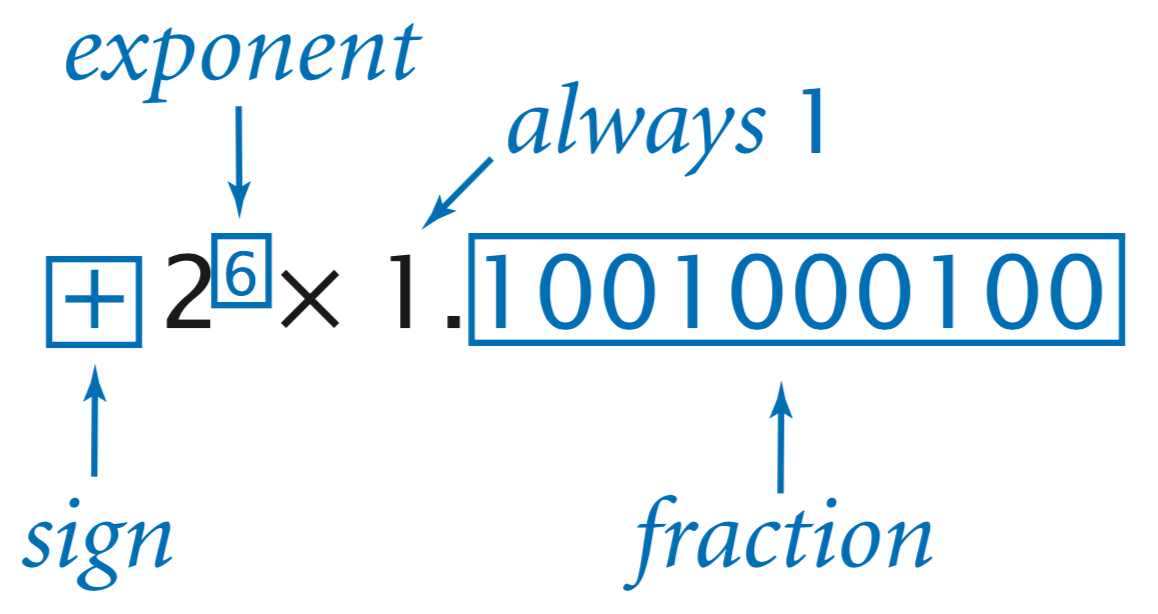• Sign. The first bit of a floating-point number is its sign. The sign bit is 0 is the number is positive (or zero) and 1 if it is negative.
• Exponent. The next t = 5 bits of a floating-point number are devoted to its exponent. The exponent of a floating-point number is expressed in offset binary, where we take R = 2t−1 – 1 (15 for binary16) and represent any decimal number x between −R and R (–15 and 16 for binary16) with the binary representation of x + R. For example, 10101 represents the exponent 6 because 6 + 15 = 21, and 101012 is the binary representation of 21.
• Fraction. The remaining 10 bits are devoted to the coefficient. The normalization condition implies that the digit before the decimal place in the coefficient is always 1, so we need not include that digit in the representation. The bits are interpreted as a binary fraction, so 1.101 corresponds to 1 + 2−1 + 2−3 = 1.625.
• Encoding and decoding floating-point numbers. Given these rules, the process of decoding a number encoded in IEEE 754 format is straightforward. The process of encoding a number is more complicated, due to the need to normalize and to extend binary conversion to include fractions.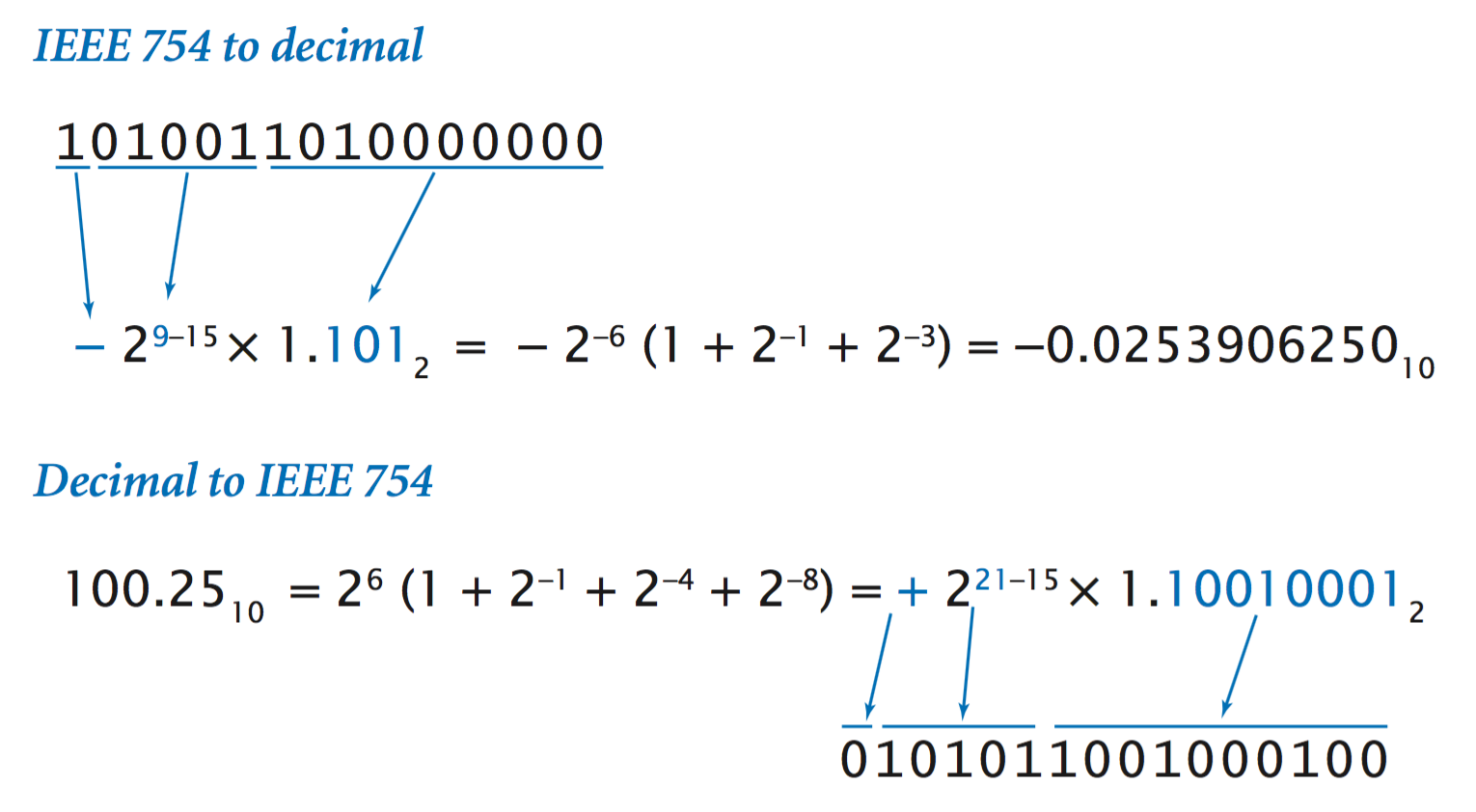• Java. Java uses binary32 for type float (32 bits, with 8 bits for the exponent and 23 bits for the fraction) and binary64 for type double (64 bits, with 11 bits for the exponent and 52 bits for the fraction). This explains the bounds on values of these types and explains various anomolous behavior with roundoff error that we first observed in Section 1.2.

## Java code for manipulating bits.

Java defines the int data type to be a 32-bit two's complement integer and support various operations to manipulate the bits.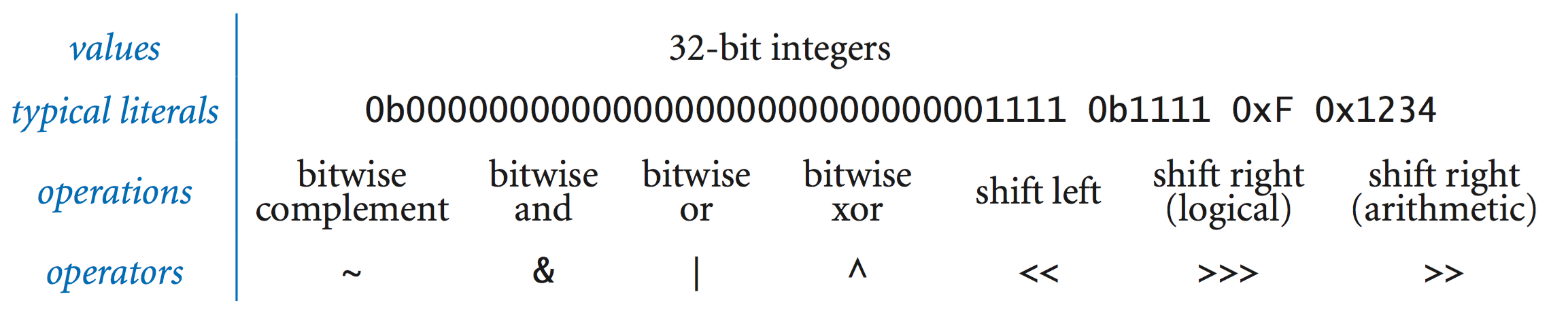Program BitWhacking.java reads in two integers a and b from the command line, applies the bit-whacking operations, and prints the results.

• Binary and hex literals. You can specify integer literal values in binary (by prepending 0b) and in hex (by prepending 0x). You can use literals like this anywhere that you can use a decimal literal.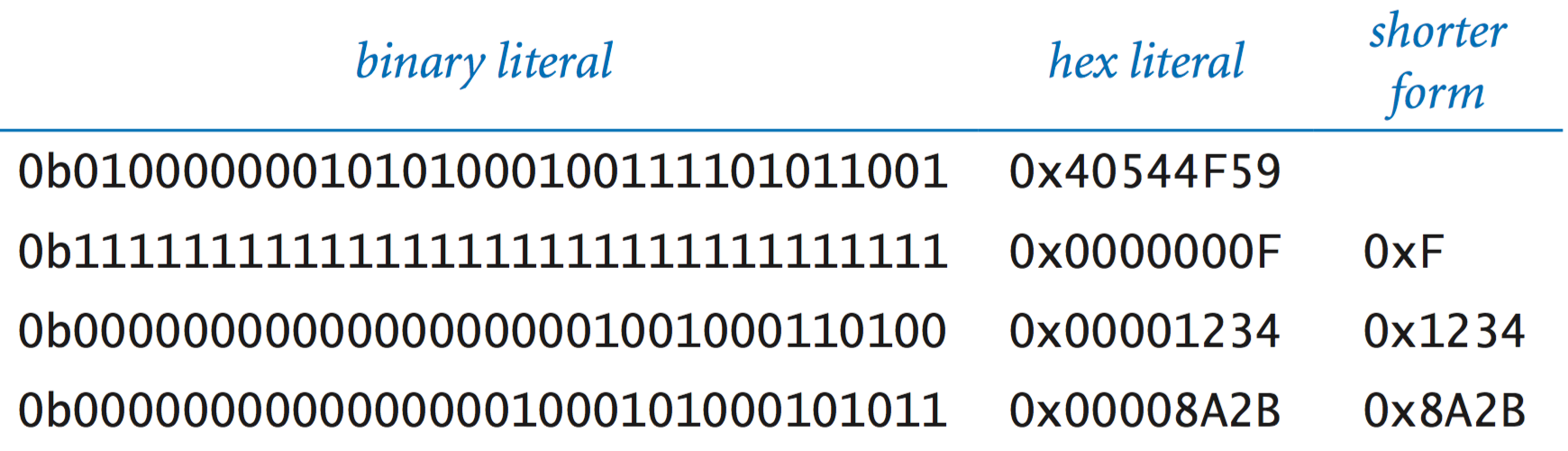• Shifting and bitwise operations. Java supports a variety of operations to manipulate the bits of an integer:
• Complement the bits: change the 0s to 1s and the 1s to 0s.
• Bitwise logical operators: apply the and, or, and exclusive or function to the corresponding pair of bits.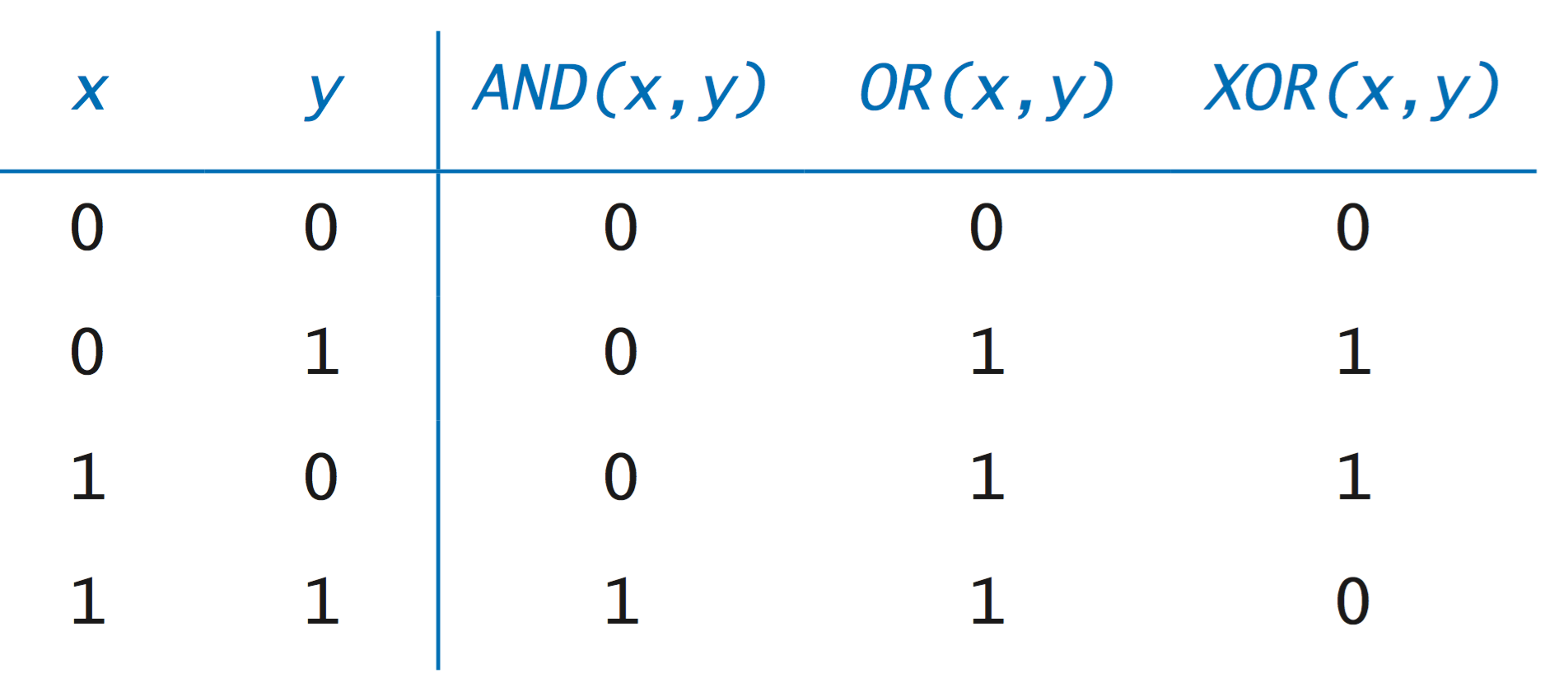• Shift left and right: shift the bits left or right a given number of positions. For shift right, there are two versions: a logical shift fills in the vacated positions at left with 0s; an arithmetic shift fills in the vacated positions at left with the sign bit.

Here are a few examples: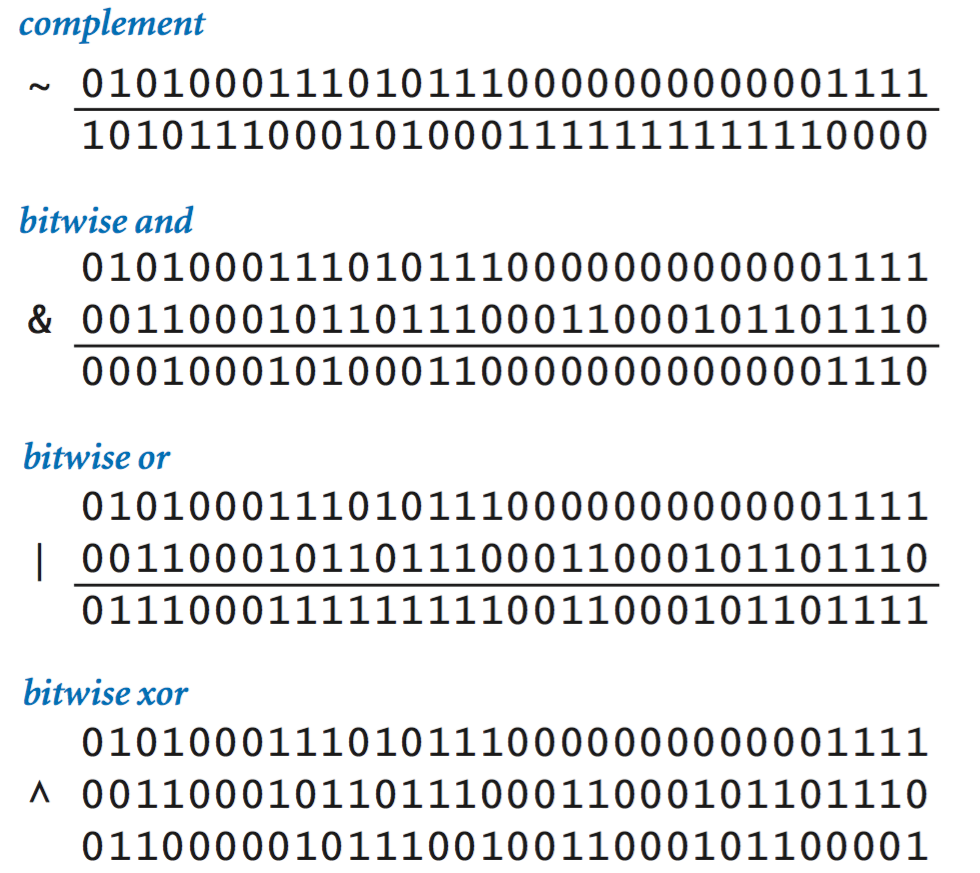• Shifting and masking. One of the primary uses of such operations is shifting and masking, where we isolate a contiguous group of bits from the others in the same word.
• Use a shift right instruction to put the bits in the rightmost position.
• If we want k bits, create a literal mask whose bits are all 0 except its k rightmost bits, which are 1.
• Use a bitwise and to isolate the bits. The 0s in the mask lead to zeros in the result; the 1s in the mask specify the bits of interest.

In the following example, we extract bits 9 through 12 from the 32-bit int.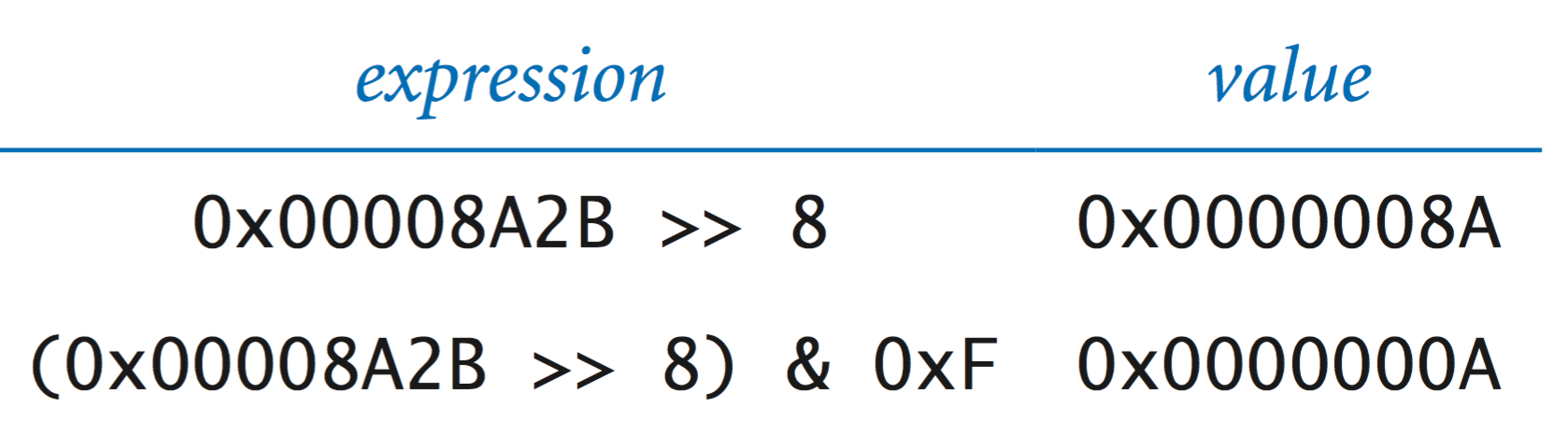ExtractFloat.java illustrates the use of shifting and masking to extract the sign, exponent, and fraction from a floating-point number.

## Characters.

To process text, we need a binary encoding for characters. The basic method is quite simple: a table defines the correspondence between characters and n-bit unsigned binary integers. ASCII and Unicode are the two most popular encoding schemes.
• ASCII. The American Standard Code for Information Interchange (ASCII) code is a 7-bit code, though in modern computing it most often is used in 8-bit bytes, with the leading bit being ignored. The following table is a definition of ASCII that provides the correspondence that you need to convert from 8-bit binary (equivalently, 2-digit hex) to a character and back. For example, 4A encodes the letter J.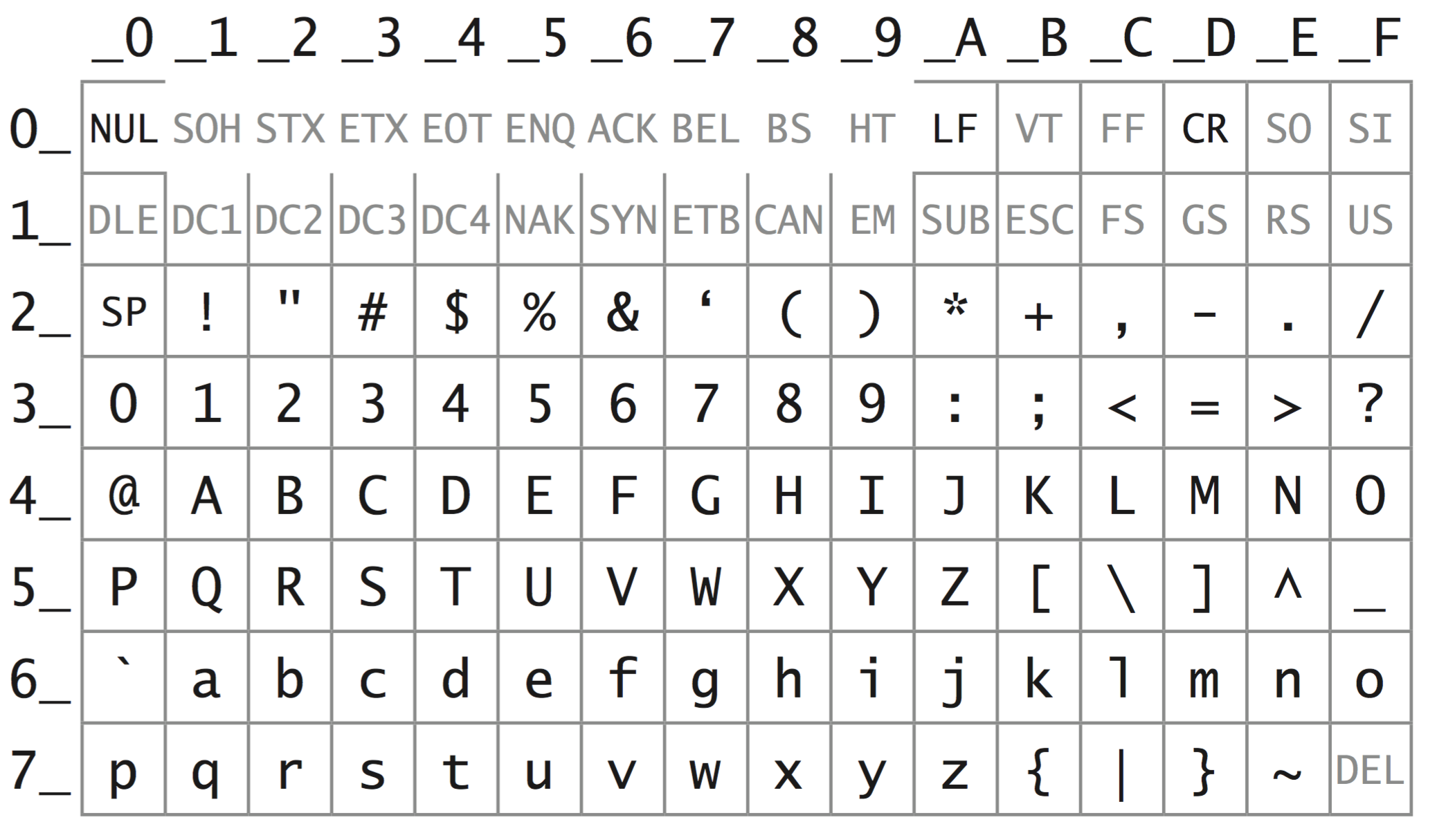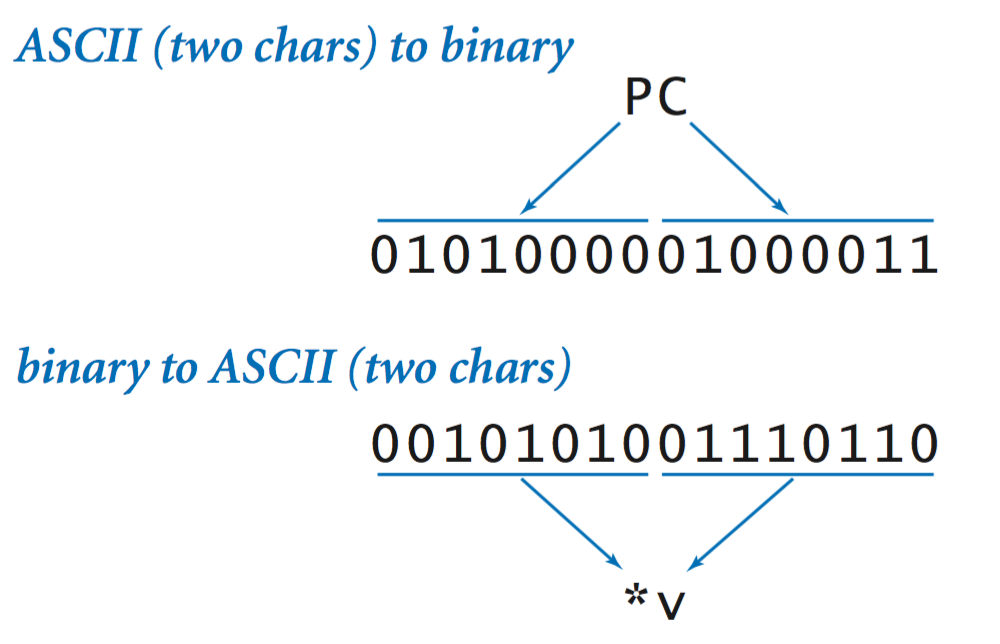• Unicode. Unicode is a 21-bit code that supports tens of thousands. The dominant implementation of Unicode is known as UTF-8. UTF-8 is a variable-width character encoding that uses 8 bits for ASCII characters, 16 bits for most characters, and up to 32 bits for other characters. The encoding rules are complicated, but are now implemented in most modern systems (such as Java) so programmers generally need not worry much about the details.

## Big Endian, little endian.

Computers differ in the way in which they store multi-byte chunks of information, e.g., the 16-bit short integer 0111000011110010 = 70F2. This consists of the two bytes 70 and F2, where each byte encodes 8 bits. The two are two primary formats, and they differ only in the order or "endianness" in which they store the bytes.
• Big endian systems store the most significant bytes first, e.g., they store the integer above in its natural order 70F2. Java uses this format, as does Apple Mac, IBM PowerPC G5, Cray, and Sun Sparc.
• Little endian systems store the least significant bytes first, e.g., they store the integer above in reverse byte-order F270. This format is more natural when manually performing arithmetic, e.g., since for addition you work from least significant byte to most significant byte. Intel 8086, Intel Pentium, Intel Xeon use this format.

#### Exercises

1. Convert the decimal number 92 to binary.

Solution: 1011100.

2. Convert the hexadecimal number BB23A to octal.

Solution: first convert to binary 1011 1011 0010 0011 1010, then consider the bits three at a time 10 111 011 001 000 111 010, and convert to octal 2731072.

3. Add the two hexadecimal numbers 23AC and 4B80 and give the result in hexadecimal. Solution: 6F2C.
4. Assume that m and n are positive integers. How many 1 bits are there in the binary representation of 2m + n? Solution: 1.
5. What is the only decimal integer whose hexadecimal representation has its digits reversed.

Solution: 53 is 35 in hex.

6. Develop an implementation of the toInt() method for Converter.java. that converts a character in the range 0-9 or A-Z into an int value between 0 and 35.
7. Develop an implementation of the toChar() method for Converter.java. that converts an int value between 0 and 35 into a character in the range 0-9 or A-Z.

#### Creative Exercises

1. IP address. Write a program IP.java that takes a 32-bit string as a command-line argument, and prints the corresponding IP address using dotted decimal notation. That is, take the bits 8 at a time, convert each group to decimal, and separate each group with a dot. For example, the binary IP address 01010000000100000000000000000001 should be converted to 80.16.0.1.

#### Web Exercises

1. Excel column numbering. Write a function that takes a nonnegative integer and converts it into the corresponding Excel column name (0 = A, 1 = B, ..., 25 = Z, 26 = AA, ..., 702 = AAA).
2. Elias Gamma coding. Write a function elias that takes as input an integer N and returns the Elias Gamma code as a string. The Elias Gamma code is a scheme to encode the positive integers. To generate the code for an integer N, write the integer N in binary, subtract 1 from the number of bits in the binary encoding, and prepend that many zeros. For example, the code for the first 10 positive integers is given below.
 1 1 6 00110 2 010 7 00111 3 011 8 0001000 4 00100 9 0001001 5 00101 10 0001010 
3. Bit reversal. Write a function that takes an integer input, reverse its bits, and returns that integer. For example if n = 8, and the input is 13 (00001101), then its reversal is 176 (10110000).
 public static int bitReverse(int input) { int ans = 0; for (int i = 0; i < n; i++) { ans = (ans << 1) + (input & 1); input = input >> 1; } return ans; } 
4. Bit-reversal sorting. Use the previous algorithm to "sort" an array of N = 2n elements into their bit-reversed order. Swap elements i and j if i and j are bit reversal of each other. Such permutations arise in the Fast Fourier Transform.
 0 1 2 3 4 5 6 12 13 14 15 0000 0001 0010 0011 0100 0101 0110 ... 1100 1101 1110 1111 0000 1000 0100 1100 0010 1010 0110 ... 0011 1011 0111 1111 0 8 4 9 2 10 6 3 11 7 15 
5. Swap without temporary storage. What do the following two code fragments do given integers a and b?
 a = a + b; b = a - b; a = a - b; a = a ^ b; b = a ^ b; a = a ^ b; 
Answer: each 3-line fragment swaps a and b. It works provided a and b are not the same variables (in which case both variables are zeroed out).
6. Find the unique integer. Suppose you have an array of 2N + 1 integers, and you know that each of N integers appear exactly twice. Describe an elegant and efficient algorithm to identify the integer that appears only once. Hint: xor.
7. Bit-whacking version of Gray codes Use bit-whacking operations and iteration instead of recursion to generate a gray code. Name your program BitWhackingGrayCode.java.
8. Free the prisoners I. A warden meets with 17 new prisoners when they arrive. The warden tells them that they may meet today and plan a strategy, but after the meeting, each prisoner will be in solitary confinement and will not be able to communicate with one another. The prison has a switch room with 17 switches that can be on or off, although the initial configuration is not revealed. There is one special setting of the 17 switches that if it is ever achieved will enable the prisoners to go free. Each hour the warden escorts one prisoner to the switch room, where the prisoner can flip at most one switch (from on to off or off to on). The warden can choose prisoners in arbitrary order, so one prisoner may be chosen four times in a row, or not at all. Design a strategy for the 17 prisoners so that they are guaranteed to be set free after some finite amount of time.
9. Free the prisoners II. Same premise as above, except that the switch room has 2 switches (initially both off), and a prisoner must flip exactly one of the two switches upon entering the switch room. At any time, a prisoner may declare "all 17 of us have visited the control room." If it is true, all prisoners are freed; otherwise they are all executed. The warden can choose prisoners in arbitrary order, so one prisoner may be chosen four times in a row, but each prisoner will be chosen infinitely often (assuming they are never freed). Design a strategy for the 17 prisoners so that they are guaranteed to be set free after some finite amount of time. Extra credit: don't assume the initial configuration is known.
10. Count the number of 1 bits. Write function that takes an integer input and returns the number of 1's in its binary representation.

Answer: here are an iterative and a recursive solution.

 public static int bitCount(int input) { int count = 0; for (int i = 0; i < 32; i++) count = count + (input >>> i & 1); return count; } public static int bitCount(int x) { if (x == 0) return 0; return (x & 1) + bitCount(x >>> 1); } 
This is how Integer.bitCount() is implemented by Java. See if you can figure out how it works.
 public static int bitCount(int i) { i = i - ((i >>> 1) & 0x55555555); i = (i & 0x33333333) + ((i >>> 2) & 0x33333333); i = (i + (i >>> 4)) & 0x0f0f0f0f; i = i + (i >>> 8); i = i + (i >>> 16); return i & 0x3f; } 
11. Sparse bit-counting. Explain why the following function (that often appears in job interviews for programmers) correctly counts the number of 1 bits in the binary representation of its input. If the input has k 1s, how many times does the while loop iterate?
 public static int bitCount(int input) { int count = 0; while (input != 0) { count++; input = input & (input - 1); } return count; } 
12. Table lookup bit-counting. Repeat the previous exercise, but pre-compute a table to speed up the computation.

Answer: this one assumes you have a precomputed table of size 256, with bits[i] storing the number of 1 bits in the binary representation of i. You can use the bit counting function from the previous exercise to initialize it. previous

 public static int bitCount(int input) { return bits[(input >> 0) & 0xff] + bits[(input >> 8) & 0xff] + bits[(input >> 16) & 0xff] + bits[(input >> 24) & 0xff]; } 

Increasing the table size to 216 = 65,536 will make things faster assuming you have sufficient memory. A table of size 232 is likely prohibitive.

13. Java library functions for bit-whacking. Re-implement the following static methods that defined in the Integer class.

FUNCTION RETURN VALUE
Integer.bitCount(x) Number of one-bits in x
Integer.highestOneBit(x) Zero out all but the leftmost one bit of x.
Integer.lowestOneBit(x) Zero out all but the rightmost one bit of x.
Integer.numberOfLeadingZeros(x) Number of zero bits preceding highest one bit.
Integer.numberOfTrailingZeros(x) Number of zero bits following lowest one bit.
Integer.rotateLeft(x, i) Rotate of x by circularly shifting i bits to the left.
Integer.rotateRight(x, i) Rotate x by circularly shifting i bits to the right.
Integer.reverse(x) Reverse of the bits of x.

14. Dictionary attack. One method that sleazy spammers use to auto-generate email addresses is by enumerating all possible email addresses at a give domain, e.g., hotmail.com. This annoying tactic is called a dictionary or Rumpelstiltskin attack and explains why you sometimes receive spam on a new email address to which you haven't given to anybody. Use Converter.java to design such a program. Your program Rumpelstiltskin.java should take a command line parameter N and print out all 36N possible passwords of N or fewer characters involving numbers and uppercase letters.
15. Breaking a gold chain. You have a gold chain with 14 links that you are going to use to pay an worker for 15 days at a fee of 1 gold link per day. It's possible to split the chain into 15 pieces by cutting 14 times. Your goal is to pay the worker while only breaking the chain 3 times. The worker must receive exactly the right fraction of total payment after each day of work. Hint: break the chain so there are pieces of 1 section, 2 sections, 4 sections, and 8 sections.
16. Hamming encoder. Write a Java program HammingEncoder.java that reads in a sequence of 0s and 1s, 4 bits at a time, and encodes them using Hamming codes.
17. Hamming decoder. Write a Java program HammingDecoder.java that reads in a sequence of 0s and 1s encoded using Hamming codes, 7 bits at a time, and decodes and correct them.
18. Hamming codes. Modify your solutions to the previous two exercises so that the input bits are packed 8 to the byte.
19. Absolute value. The constant Integer.MIN_VALUE is the most negative 32-bit two's complement integer. What is Math.abs(Integer.MIN_VALUE)?
20. Prove that a k-digit decimal number can be represented in binary with no more than 4k bits.
21. Sum of powers of 2. Compute the sum of powers of 2. What value do you end up with on two's complement machine?
22. CD Database. CDDB and freedb are databases that allow you to look up CD information on the Web and display the artist, title, and song name. Each CD has a (nearly) unique disc ID number which is used to query the database.
1. Write a static method sumDigits() that takes an integer parameter and returns the sum of the decimal digits in the integer. For example, sumDigits(6324) returns 15 since 6 + 3 + 2 + 4 = 15.
2. Write a program CDDB.java that computes the disc ID from a list of lengths of the track lengths. The 32-bit (8 hex digit) ID number is computed from the length of the tracks on the CD and the number of tracks as follows:
 XXYYYYZZ XX = checksum of track offsets in seconds, taken mod 255 YYYY = length of the CD in seconds ZZ = number of tracks on the CD 
23. True or false. If a xor b = c, then c xor a = b and c xor b = a.
24. Explain why the following code fragment does not leave ABCD in variable a. How would you fix it?
 byte b0 = 0xAB; byte b1 = 0xCD; int c = (b0 << 8) | b1; 
Answer. In Java, byte is a signed 8-bit integer. The right-shift promotes b0 to a (negative) integer. To fix the problem, use c = ((b0 & 0xff) << 8) | (b1 & 0xff);.
25. Poisonous wine. "You are the ruler of an empire and you are about to have celebration tomorrow. The celebration is the most important party you have ever hosted. You've got 1000 bottles of wine you were planning to open for the celebration, but you find out that one of them is poisoned. The actual poison exhibits no symptoms until somewhere around the 23rd hour, then results in sudden death. You have thousands of prisoners at your disposal. What is the smallest number of prisoners you must have to drink from the bottles to find the poisoned bottle?"

Hint: you can represent the number 1,000 using 10 bits.

26. Unsigned 32-bit integers. Describe how to simulate 32-bit unsigned integers in Java.

Solution: First, are you sure that you really need an unsigned type. Signed and unsigned integers behave identically on the bitwise operators (except >>), addition, subtraction, and multiplication. In many applications, these are sufficient, assuming you replace >> with >>>. Comparison operators are easy to simulate by checking the sign bit. Division and remainder are the trickiest: the easiest solution is to convert to type long.

 long MASK = (1L << 32) - 1; // 0x00000000FFFFFFFF; int quotient = (int) ((a & MASK) / (b & MASK)); int remainder = (int) ((a & MASK) % (b & MASK)); 
Program UnsignedDivision.java uses this trick, and also does it directly using 32-bit operations.
27. Unsigned 8-bit integers. Describe how to simulate 8-bit unsigned integers in Java.

Solution: Same advice as previous question. One place where it's nice to have unsigned integers is for a lookup table, indexed by the byte. With signed integers the index can be negative. Also, if b is a byte, then b << 4 automatically casts b to an int. This could be undesirable since b is signed. In many applications you need to remove the signed extended bits via (b << 4) & 0xff.

28. Adding two short integers. Explain why the following code fragment fails.
 short a = 4; short b = 5; short c = a + b; 
Solution: Java automatically promotes the results of most integer operations to be of type int. To assign the result to a short, you need to explicitly cast it back c = (short) (a + b). Yes, this is rather quirky. One exception to this rule is if you use +=, in which case the cast is performed automatically.

Masking with byte. Explain why (b << i) give weird results when b is of type byte.

Solution: In Java, byte is an 8-bit signed integer. Before the right shift, b is converted to an integer. You may want ((b & 0xff) << i) instead.

29. How many bits are in the binary representation of 2^2^2^2^17?
30. What does the following code fragment from program Overflow.java print?
 int a = 2147483647; // 2^31 - 1 int b = a + 1; System.out.println("a = " + a); System.out.println("b = " + b); 
31. What does the following code fragment print?
 int a = -5 >> 3; int b = -5 >>> 3; System.out.println(a); System.out.println(b); 
32. List all values a of type int for which (a == (a >> 1)). Hint: there is more than one.
33. Suppose a is a variable of type int. Find two values of a for which (a == -a) is true. Answer: 0 and -2147483648.
34. What is the result of a = -1 * -2147483648? Answer: 0.
35. What does the following code fragment print out?
 int a = 11 & 17; int b = 11 ^ 17; int c = 11 | 17; int d = ~11; System.out.println(a); System.out.println(b); System.out.println(c); System.out.println(d); 
36. Given two positive integers a and b, what result does the following Java code fragment leave in c?
 c = 0; while (b > 0) { if (b & 1 == 1) c = c + a; b = b >> 1; a = a << 1; } 

37. What does the following code do to the integers stored in two different variables a and b?
 a = a ^ b; b = a ^ b; a = a ^ b; 
38. Repeat the previous question, but assume a and b are the same variable.
39. What does the following code do to the integers stored in two different variables a and b? Any problems with overflow?
 a = a + b; b = a - b; a = a - b; 
40. What do each of the following statements do?
 x = - ~x; x = ~ -x; 

Increment x, decrement x

41. What does the following do?
 public static boolean parity(int a) { a ^= a >>> 32; a ^= a >>> 16; a ^= a >>> 8; a ^= a >>> 4; a ^= a >>> 2; a ^= a >>> 1; return a & 1; } 
Answer: computes the parity of the number of 1 bits set in the binary representation of a using divide-and-conquer.
42. What is the value of cnt after the following loop?
 int cnt = 0; for (int i = 1; i != 0; i = 2 * i) { cnt++; } 
Hint: it's not an infinite loop.
43. Explain why the following Java code fragment correctly determines whether the integer n is a power of 2.
 boolean isPowerOfTwo = (n & -n) == n; 
44. Counting in base -2. Use the definition of the positional notation to define the base -2 number system. There are two digits 0 and 1. Count from -7 to 7 in this system.
 0 = 0 -1 = 11 (-2 + 1) 1 = 1 -2 = 10 2 = 110 (4 + -2) -3 = 1101 (-8 + 4 + 1) 3 = 111 -4 = 100 4 = 100 -5 = 1111 5 = 101 -6 = 1110 6 = 11010 (16 + -8 + -2) -7 = 1001 7 = 11011 
45. RGBA color format. Some of Java's classes (BufferedImage, PixelGrabber) use a special encoding called RGBA to store the color of each pixel. The format consists of four integers, representing the red, green, and blue intensities from 0 (not present) to 255 (fully used), and also the alpha transparency value from 0 (transparent) to 255 (opaque). The four 8-bit integers are compacted into a single 32-bit integer. Write a code fragment to extract the four components from the RGBA integer, and to go the other way.
 // extract int alpha = (rgba >> 24) & 0xff; int red = (rgba >> 16) & 0xff; int green = (rgba >> 8) & 0xff; int blue = (rgba >> 0) & 0xff; // write back rgba = (alpha << 24) | (red << 16) | (green << 8) | (blue << 0); System.out.println(rgba); 
46. Min and max. One of the following computes min(a, b), the other computes max(a, b) without branching. Which is which? Explain how it works.
 f = b + ((a - b) & -(a < b)); // min(a, b) g = a - ((a - b) & -(a < b)); // max(a, b) 
47. Find the missing value. Suppose you have an array consisting of 232 - 1 integers of type int such that no integer appears more than once. Since there are 232 possible values, exactly one integer is missing. Write a code fragment to find the missing integer using as little extra storage as possible.

Hint: this is a popular interview question. It's possible to do it using only one extra int. Use either properties of integer overflow on two's complement integers or use the XOR function.

48. Cyclic redundancy check. Write programs CRC16.java and CRC32.java that read in data from standard input and computes its 16 or 32-bit CRC. Write a program CRC16CCITT.java" for 16-bit CRC in CCITT format.